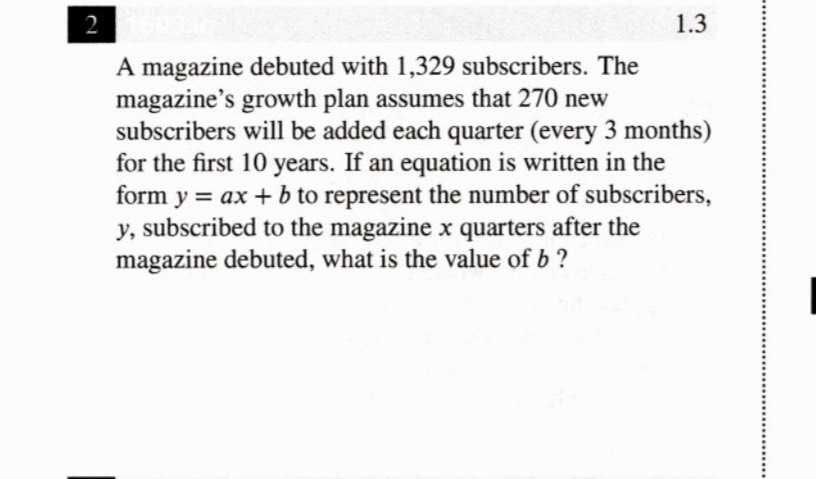### ¿Todavía tienes preguntas de matemáticas?

Pregunte a nuestros tutores expertos
Algebra
PreguntaA magazine debuted with $$1,329$$ subscribers. The magazine's growth plan assumes that $$270$$ new subscribers will be added each quarter (every $$3$$ months) for the first $$10$$ years. If an equation is written in the form $$y = a x + b$$ to represent the number of subscribers,

$$y$$ , subscribed to the magazine $$x$$ quarters after the magazine debuted, what is the value of $$b$$ ?